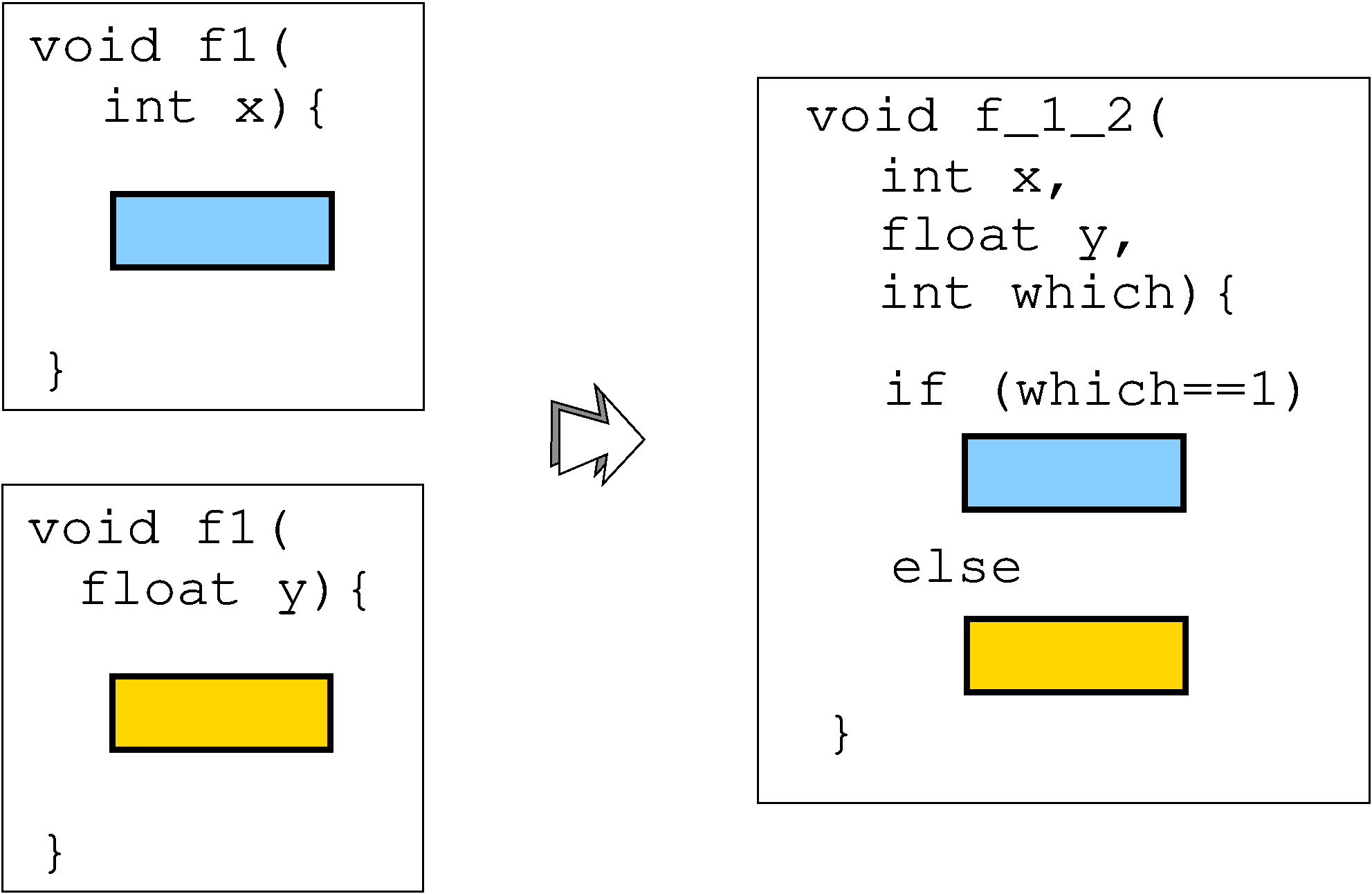Merge multiple functions into one. An extra formal argument is added to allow call sites to call any of the functions. This transformation is useful as a precursor to virtualization or jitting: if you want to virtualize both foo and bar, first merge them together, then virtualize the result.The transformation merges the argument list and the local variables of the functions, thereby tying them together.

### Diversity

Merging relies on the Flatten transformation, and has the same sources of diversity as it.

### Usage

There are several ways to merge. In a simple merge, the function bodies are simply put in an if-nest. This is simplistic, of course, but sufficient if you are going to, say, virtualize or jit the merged function. If you set --MergeFlatten=true then constituent functions are first flattened, then the resulting blocks are merged together, and finally a dispatch method is added (switch, goto, or indirect, selected by --MergeFlattenDispatch).

The merged function is named

```   prefix ^ fun1 ^ "_" ^ fun2  ^ "_" ^ ...
```

where ^ is concatenation.

It is a good idea to run a --Trandform=RndArgs transformation after this one to hide the obvious extra argument that's been added to the function.

### Options

OptionArgumentsDescription
--Transform Merge Merge of two or more functions. Two different types of merge are supported: simple merge (if () function1 else if () function2 else ...) and flatten merge, where the functions are first flattened, and then the resulting blocks are woven together. This transformation modifies the signature of the function (an extra formal selector argument is added that selects between the constituent functions at runtime), and this cannot be done for functions whose address is taken. --Functions=\* merges together all functions in the program whose signatures can be changed, --Functions=%50 merges together about half of them, etc. It is a good idea to follow this transform by a RndArgs transform to hide the extra selector argument.
--MergeName string If set, the merged function will be named prefix_name, otherwise it will be named prefix_originalName1_originalName2. Note that it's unpredictable which function will be the first and the second, so it's better to set the merged named explicitly.
--MergeObfuscateSelect BOOLSPEC Whether the extra parameter passed to the merged function should be obfuscated with opaque expressions or not. Default=false.
--MergeOpaqueStructs list, array, * Type of opaque predicate to use. Traditionally, for this transformation, array is used. Default=array.
• list = Generate opaque expressions using linked lists
• array = Generate opaque expressions using arrays
• * = Same as list,array
--MergeFlatten BOOLSPEC Whether to flatten before merging or not. Default=true.
--MergeFlattenDispatch switch, goto, indirect, ? Dispatch method used for flattened merge. Default=switch.
• switch = dispatch by while(1) {switch (next) {blocks}}
• goto = dispatch by {labl1: block1; goto block2;}
• indirect = dispatch by goto* (jtab[next])
• ? = select an dispatch method at random.
--MergeSplitBasicBlocks BOOLSPEC If true, then basic blocks (sequences of assignment and call statements without intervening branches) will be split up into indiviual blocks prior to merging. Default=false.
--MergeRandomizeBlocks BOOLSPEC If true, then basic block sequences will be randomized. Default=false.
--MergeConditionalKinds branch, compute, flag If merging before flattening, this option describes ways to transform conditional branches. Default=branch.
• branch = Use normal branches, such as if (a>b) goto L1 else goto L2
• compute = Compute the branch, such as x=(a>b); goto *(expression over x)
• flag = Compute the branch from the values of the flag register, such as asm("cmp a b;pushf;pop"); goto *(expression over flag register)

### Examples

Merge
Merge fib and fac into fac_fib.
```tigress --Environment=x86_64:Darwin:Clang:5.1 --Verbosity=1  \
--Transform=InitEntropy --Functions=main \
--Transform=InitOpaque --Functions=main --InitOpaqueCount=2 --InitOpaqueStructs=list,array \
--Transform=Merge --Functions=fac,fib \
--Transform=CleanUp --CleanUpKinds=annotations \
--out=gen/merge.c test1.c
```
test1.cmerge.sh.txtmerge.c

Merge ⇒ Split
Merge fac and fib into fac_fib, and then split up fac_fib.
```tigress --Environment=x86_64:Darwin:Clang:5.1 --Verbosity=1  \
--Transform=InitEntropy --Functions=main \
--Transform=InitOpaque --Functions=main --InitOpaqueCount=2 --InitOpaqueStructs=list,array \
--Transform=Merge --Functions=fac,fib --MergeName=MERGED \
--Transform=Split --SplitKinds=block,top,deep --SplitCount=10 --Functions=MERGED \
--Transform=CleanUp --CleanUpKinds=annotations \
--out=gen/merge-split.c test1.c
```
test1.cmerge-split.sh.txtmerge-split.c

Merge ⇒ Flatten
Merge fac and fib into fac_fib, and then flatten fac_fib.
```tigress --Environment=x86_64:Darwin:Clang:5.1 --Verbosity=1  \
--Transform=InitEntropy --Functions=main \
--Transform=InitOpaque --Functions=main --InitOpaqueCount=2 --InitOpaqueStructs=list,array \
--Transform=Merge --Functions=fac,fib --MergeName=MERGED \
--Transform=Flatten --Functions=MERGED \
--Transform=CleanUp --CleanUpKinds=annotations \
--out=gen/merge-flatten.c test1.c
```
test1.cmerge-flatten.sh.txtmerge-flatten.c
Merge
Merge fac and fib into fac_fib, but first flatten them.
```tigress --Environment=x86_64:Darwin:Clang:5.1 --Verbosity=1  \
--Transform=InitEntropy --Functions=main \
--Transform=InitOpaque --Functions=main --InitOpaqueCount=2 --InitOpaqueStructs=list,array \
--Transform=Merge --Functions=fac,fib --MergeFlatten=true --MergeFlattenDispatch=indirect \
--Transform=CleanUp --CleanUpKinds=annotations \
--out=gen/merge_flatten.c test1.c
```
test1.cmerge_flatten.sh.txtmerge_flatten.c
Merge ⇒ Flatten ⇒ RndArgs ⇒ Virtualize ⇒ AddOpaque ⇒ Split
Merge fac and fib, flatten, add bogus arguments, replace literals with opaque expressions, virtualize, split up control flow with opaque predicates, and split up the resulting function.
```tigress --Environment=x86_64:Darwin:Clang:5.1 --Verbosity=1  \
--Transform=InitOpaque --Functions=main \
--Transform=Merge --Functions=fac,fib \
--Transform=Flatten --Functions=/.\*fac_fib.\*/ \
--Transform=RndArgs --RndArgsBogusNo=2?5 --Functions=/.\*fac_fib.\*/ \
--Transform=EncodeLiterals  --Functions=/.\*fac_fib.\*/ \
--Transform=Virtualize --Functions=/.\*fac_fib.\*/ --VirtualizeDispatch=ifnest \
--Transform=UpdateOpaque --Functions=/.\*fac_fib.\*/ --UpdateOpaqueCount=10 \
--Transform=Split --Seed=0 --SplitKinds=deep,block,top --SplitCount=100 --Functions=/.\*fac_fib.\*/ \
--Transform=CleanUp --CleanUpKinds=annotations \
--out=gen/merge-flatten-virtualize.c test1.c

```
test1.cmerge-flatten-virtualize.sh.txtmerge-flatten-virtualize.c

### Issues

• Consider this example taken from gcc's comp-goto-1.c torture test:
```goto *(base_addr + insn.f1.offset);
```
This kind of arithmetic on the program counter is going to fail for transformations that completely restructure the code, such as --Transform=Merge --MergeFlatten=true.
• The --MergeConditionalKinds=flag option seems to have multiple issues on MacOS/llvm. Presumably this is due to some compiler problem related to inline assembly.

### References

• I believe merging of flattened functions first appears in Chenxi Wang's thesis.